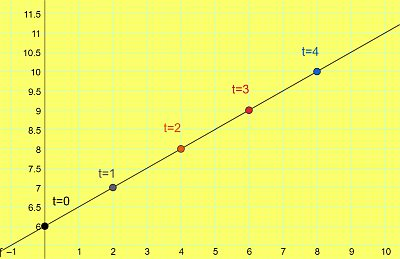# Sketch the graph of x= 2t, y= t + 6.

## Question:

Sketch the graph of {eq}x= 2t, y= t + 6. {/eq}

## Parametric Equations:

Parametric equations are the relations between two variables with the help of a third variable, which we call a parameter. To graph a parametric equation, we usually take several values of a parameter {eq}t {/eq} and put those values to get the values of our variables. Parametric representation often helps to simplify complex looking functions.

To graph {eq}x {/eq} and {eq}y {/eq} what we'll need is a set of points. The set of points which we choose for some values of the parameter {eq}t {/eq} are as follows...

Sr No. 1 2 3 4 5
x 0 2 4 6 8
y 6 7 8 9 10
t 0 1 2 3 4

So, the set of points that we get from the table above are {eq}(0,6),(2,7),(4,8),(6,9),~\&~(8,10) {/eq}

Let's graph them ...Check: We see that the parametric relation defined in the question, gives us a line as shown in the graph. What would the equation of that line be?

Let's put {eq}t=y-6 {/eq} in the {eq}x=2t {/eq}.

{eq}\therefore{x=2(y-6)}\\ \therefore{x}=2y-12\\ \therefore{2y-x=12} {/eq}

So, {eq}2y-x=12 {/eq} is the required line.